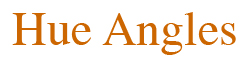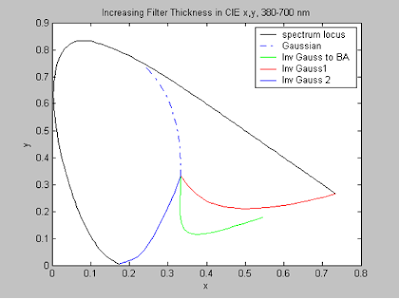## Monday, June 30, 2008

### Beer's-law dyes and the purple limit

by Michael H. Brill, Datacolor

Everyone who takes a colorimetry course learns that, when you pile on layers of a light-transmitting material (multiplying the transmission spectrum by itself, in an action called Beer’s law), the transmitted light gets dimmer and more nearly monochromatic, and its chromaticity approaches the spectrum locus. The limiting wavelength on the spectrum locus is the maximum-transmission wavelength of the original (unit-thickness) transmission curve. Starting from that idea, how can we design a Beer's-law-unit-thickness transmission curve for a material such that greater and greater thickness of the material, trans-illuminated by the same light, would approach arbitrarily closely to a given chromaticity point on the line of purples?

Here's how. First, design a U-shaped transmission curve with its two maxima at the ends of the visible spectrum. As you multiply this spectrum by itself (i.e., increase its thickness), the bigger maximum will eventually dominate and the chromaticity will go to the blue or red end of the spectrum---the end with the global maximum of the U. So far we have reached (arbitrarily closely) only two points on the line of purples.

Now design a U-shaped transmission curve with equal maxima at the ends of the spectrum. As you multiply this spectrum by itself, neither maximum ever dominates, so the chromaticity must move to a mid value on the line of purples (whose location depends on the illuminant spectrum at the endpoint wavelengths of the visible spectrum). This is what I call the "Buridan's ass" (or BA) point, named after the donkey invented by Jean Buridan (1300-1358) that starved to death when placed exactly equidistant from two bales of hay. For the transmission curve, the analogue of starvation is balance between the spectrum-locus ends.

Finally, design a U-shaped transmission curve with maxima that are different by only, say, one part in 1010. As you multiply this spectrum by itself, initially neither maximum dominates, so the chromaticity moves toward the BA point. But eventually, that 1 part in 1010 breaks the symmetry, and the transmission rapidly moves, from (very) near the line of purples at the BA point, to one end of the spectrum. A single dye can be made, by self-multiplication, to come arbitrarily close to all the purple-line points on one side of the BA point. Another dye with a similar imbalance the other way will do the same for the other side of the BA point. QED.

Let’s simulate the filter algebra using Gaussians and inverse Gaussians, equal-energy illuminant, and 1931 CIE color-matching functions. Figure 1 shows the unit-thickness transmission spectra, and Fig. 2 shows the chromaticity trajectories of these spectra as thickness is increased. The BA point is given by

XBA = [ X(λe) + X(λb)] / [X(λe) + X(λb) + Y(λe) + Y(λb) + Z(λe) + Z(λb)]
YBA = [ Y(λe) + Y(λb)] / [X(λe) + X(λb) + Y(λe) + Y(λb) + Z(λe) + Z(λb)].

Here, λb and λe are the wavelengths at the beginning and end of the visible spectrum. I chose λb = 380 nm and λe = 700 nm, so BA is at (0.5458, 0.1776).

Note that the BA point is sensitive to the choice of λb and λe. If I change λe to 720 nm, then BA moves to (0.3615, 0.0920). Amazingly, the chromaticity at λe stays the same to five decimal places!Figure 1.Figure 2.

After reading this essay, Jack Ladson asked me, “Did Buridan recognize that repeated multiplication of the spectrum ends in black?” I'm sure Buridan, like other philosophers, recognized analogously that all life ends in death, but it matters from which direction you enter that state. In both cases, given enough light, you can see the direction.

Question for discussion: For a real filter, does Beer’s law break down so the chromaticity of a light shone through increasing thickness of the filter fails to approach the spectrum locus?